Previous Chapter

## Competitive Equilibrium in a Simple Economy

### The Consumer: Choice and Indifference Curves

PRICE, COST, AND VALUE

A very old puzzle in economics is the relation between price, value to the consumer, and cost of production. It is tempting to say that the price of a good is determined by its value to the user. Why, after all, would anyone buy a good for more or sell it for less? But if this is so, why are diamonds, which are relatively unimportant (most of us could get along quite well if they did not exist), worth so much more per pound than water, which is essential for life? If the answer is that diamonds are rare and that it is rarity rather than usefulness that determines price, I reply that signatures of mine written in yellow ink are even rarer than original autographs of Abraham Lincoln but bring a (much) lower price.

Perhaps it is cost of production that determines price. When I was very young, I used to amuse myself by shooting stalks of grass with a BB gun. That is a costly way of mowing the lawn, even considering that the cost per hour of a nine-year-old's time is not very high. I think it unlikely that anyone would pay a correspondingly high price to have his lawn mowed in that fashion.

This puzzle--the relation between value to the consumer, cost of production, and price--was solved a little over 100 years ago. The answer is that price equals both cost of production and value to the user, both of which must therefore be equal to each other. How market mechanisms arrange that triple equality will be discussed in the next few chapters. In this chapter and the next, we shall analyze the behavior of a consumer who must decide what to buy with his limited resources; among the things we shall learn in the process is why, as a consequence of rational behavior by the consumer, price equals (marginal) value.

LANGUAGES

There are several different languages in which the problem of consumer behavior--and many other problems in economics--can be analyzed. Each of these languages has advantages and disadvantages. One may use the language of calculus, making assumptions about the form of the "utility function" that describes the individual's preferences among different goods and deducing the characteristics of the bundle of goods that maximizes it. This has the advantage of allowing compact and rigorous mathematical arguments and of producing very general results, applicable to a wide range of possible situations. It has the disadvantage that even if you know calculus, you probably do not know it in the same sense in which you know English. Unless you are very good at intuiting mathematics, you can follow a proof step by step from assumptions to conclusions and still not know why the result is true. For these reasons, calculus and utility functions will be used only in some of the optional sections of this text. The ordinary sections will not assume any knowledge of either, although a few concepts borrowed from calculus will be explained in simple terms and used where necessary.

Another possible language is geometry. Most of us can understand abstract relations better as pictures than as equations; hence geometric arguments are easier to intuit. One disadvantage of geometry is that it limits us to situations that can be drawn in two dimensions--typically, for example, to choices involving only two different goods. A second disadvantage is that we may, in drawing the picture, inadvertently build into it assumptions about the problem--possibly false ones.

The third language is English. While not as good as mathematical languages for expressing precise quantitative relations, English has the advantage of being, for most of us, our native tongue. Insofar as we think in words at all, it is the language we are used to thinking in. Unless we have very good mathematical intuition, all mathematical arguments eventually get translated, in our heads, into words, and it is only then that we really understand them. Alfred Marshall, possibly the most important economist of the past century, wrote that economic ideas should be worked out and proved in mathematical form and then translated into words; if you find that you cannot put your analysis into words, you should burn your mathematics. Since it is often hard to keep track of quantitative relations in a verbal argument, explanations given in English will frequently be supplemented by tables.

This chapter presents the logic of a consumer, first in verbal form, then in a simple geometrical form suitable for describing the choice between two different goods. The analysis is continued in the next chapter, which uses a somewhat more complicated geometric argument, designed to produce calculus results without actually using calculus. Among the results are the answers to three interesting questions: How does the amount you buy depend on price? How much do you benefit by being able to buy something at a particular price? What is the relation between price and value?

THE CONSUMER I: ENGLISH VERSION

Your problem as a consumer is to choose among the various bundles of goods and services you could purchase or produce with your limited resources of time and money. There are two elements to the problem--your preferences and your opportunity set. Your preferences could be represented by a gigantic table showing all possible bundles--collections of goods and services that you could conceivably consume--and showing for every pair of bundles which one you prefer. We assume that your preferences are consistent; if you prefer A to B and B to C, you also prefer A to C. Your opportunity set can be thought of as a list containing every bundle that you have enough money to buy. Your problem as a consumer is to decide which of the bundles in your opportunity set you prefer.

I will simplify the problem in most of this chapter by considering only two goods at a time--in this part of the chapter, apples and oranges. We may imagine either that these are the only goods that exist or else that you have already decided how much of everything else to consume. We assume that both apples and oranges are goods, meaning that you prefer more to less. Things that are not goods are either neutral (you do not care how much you have) or bads (you prefer less to more: garbage, strawberry ice cream, acid rock). As these examples suggest, whether something is a good or a bad for you depends on your preferences; some people like strawberry ice cream.

#### Preferences: Patterns on a Table

Table 3-1
 Bundle Apples Oranges Utility Bundle Apples Oranges Utility A 10 0 5 F 2 8 5 B 7 1 5 G 10 1 6 C 5 2 5 H 8 2 6 D 4 3 5 J 7 3 6 E 3 5 5 K 9 1 ? L 7 5 ?

Table 3-1 is a list of bundles of apples and oranges. For each bundle, the table shows its name (A-L), how many apples and oranges it contains, and its utility--an abstract measure of how much you value the bundle. The statement "Bundle A and bundle C have the same utility" is equivalent to the statement "Given a choice between A and C, you would not care which you got." The statement "Bundle G has greater utility than bundle B" is equivalent to "Given a choice between B and G, you would choose G." Listing a utility for each bundle is a simple way of describing your preferences; by comparing the utilities of two bundles, we can see which you prefer.

Utility is being used here as an ordinal measure--the order matters (bundle G has more utility than bundle F, so you prefer G to F) but the amount does not. In Chapter 13, we will expand the idea of utility in a way that converts it into a cardinal measure--one for which both order (bundle G has more utility than bundle F) and size (bundle G has 1 utile more utility than bundle F) matter. Since in this chapter, utility describes the choices of one individual, we need not worry about interpersonal utility comparisons--questions such as "Does an orange have more utility to me or to you?" We will say a little more about that question in Chapter 15, when we are trying to evaluate changes that make some people better off and some worse off.

Since bundles A-F have the same utility (5), you are indifferent among them. If you started with 4 apples and 3 oranges (D) and somehow gained an apple and lost an orange, moving from D to C, you would be neither better nor worse off. We will say that in such a situation an apple and an orange have the same value to you, or alternatively, that the value of an apple is 1 orange; the value of an orange is 1 apple.

It is important to note that the statement "The value of an apple is 1 orange" is true only between C and D. As we move up or down the table, values change. If you start with 5 apples and 2 oranges, you must receive not 1 but 2 apples to make up for losing 1 orange; in this situation (between B and C), the value of an orange is equal to that of 2 apples. An orange is worth 2 apples; an apple is worth half an orange.

The numbers in bundles A-F follow a pattern--as you move up the table, it takes more and more apples to equal 1 orange; as you move down, it takes more and more oranges to equal 1 apple. The numbers are set up this way because, as a rule, the more you are consuming of something, the less you value consuming one more. If you have very few oranges, you will be willing to give up a good deal to have one more (assuming you like oranges). If you are already consuming 12 oranges per day, you will be willing to give up very little to have 13 instead. As we move up the column, each successive bundle has fewer oranges and more apples, so in each successive case oranges are worth more to you and apples less, making each orange worth more apples. This general pattern is referred to as a declining marginal rate of substitution --the rate at which additional apples substitute for additional oranges declines with increases in the number of apples or decreases in the number of oranges.

Another way of seeing the pattern is to ask how many oranges it takes to raise your utility by 1. If you start at A, the answer is that it takes 1 orange; adding 1 orange to your bundle puts you at G, with a utility of 6, up 1 from 5. If you start at B, it takes 2 extra oranges to move you to J, increasing your utility by 1. At B you already have 1 orange, so the extra utility you get from an additional orange (the marginal utility of an orange) is less than at A, where you start with no oranges at all.

The name for this pattern is the principle of declining marginal utility--marginal utility because what is declining as you have more and more oranges is the additional utility to you of having one more orange. It is the same principle that was introduced in the previous chapter when I discussed why I would not trade my life for any quantity of Baskin-Robbins ice cream cones. Figure 2-1 showed that as the rate at which I consumed ice cream cones increased, the additional utility from each additional cone became less and less. Eventually I reached a rate of consumption at which increased consumption resulted in decreased utility--the additional utility from additional ice cream cones was negative.

#### Trading toward an Optimal Bundle

Suppose you start with bundle A on Table 3-1, and someone offers to trade oranges for your apples at a rate of 1 for 1. You accept the offer, and trade 1 apple for 1 orange. That gives you bundle K. Since K has more apples than B and as many oranges, you prefer K to B; since B is equivalent to A, you prefer K to A. We do not know what K's utility is, but it must be more than 5 (and less than 6. Why?).

To figure out how many apples you would be willing to exchange for oranges at a rate of 1 for 1, we would need to add many more bundles to the table. That problem is more easily solved using the geometric approach, which will be introduced in the next part of the chapter. There are, however, a number of lessons that can be drawn from this rather simple analysis of consumer choice.

The first is that the value of something is whatever we are (just) willing to give up for it. Two things have the same value if gaining one and losing the other leaves us neither better nor worse off--meaning that we are indifferent between the situation before the exchange and the situation after the exchange. This is an application of the principle of revealed preference discussed in the previous chapter--our values are defined by the choices we make.

A second lesson is that the value of goods (to you) depends not only on the nature of the goods and your preferences but also on how much of those goods you have. If you have 1 apple and 12 oranges, an orange will be worth very little (in apples). If you have 10 apples and no oranges, an orange is worth quite a lot of apples--3, according to Table 3-1.

The third lesson is that the price (or cost) of a good is the amount of something else you must give up to get it. In our example, where someone is willing to trade oranges for apples at a rate of 1 for 1, the price of an apple is 1 orange and the price of an orange is 1 apple. This is called opportunity cost--the cost of getting one thing, whether by buying it or producing it, is what you have to give up in order to get it. The cost of an A on a midterm, for example, may turn out to be three parties, two football games, and a night's sleep. The cost of living in a house that you already own is not, as you might think, limited to expenditures on taxes, maintenance, and the like; it also includes the interest you could collect on the money you would have if you sold the house to someone else instead of living in it yourself.

Opportunity cost is not a particular kind of cost but rather the correct way of looking at all costs. The money you spend to buy something is a cost only because there are other things you would like to spend the money on instead; by buying A, you give up the opportunity to buy B. Not getting the most valuable of the B's that you could have bought with the money--the one you would have bought if A had not been available--is then the cost to you of buying A. That is why, if you were certain that the world was going to end at midnight today, money would become almost worthless to you. Its only use would be to be spent today--so you would "spend as if there were no tomorrow."

The final lesson is that you buy something if and only if its cost is less than its value. In the example we gave, the cost of an orange was 1 apple. The value of an orange, between bundles A and B, was 3 apples. So you bought it. That put you at bundle K. If, starting from there, the value of an orange was still more than 1 apple, you would have bought another. As you trade apples for oranges, the number of apples you have decreases and the number of oranges increases. Because of the principle of declining marginal utility, additional oranges become less valuable and apples become more valuable, so the value of (one more) orange measured in apples falls. When, as a result of trading, you reach a bundle for which the value of yet another orange is no more than its price, you stop trading; you have reached the best possible bundle, given your initial situation (bundle A) and the price at which you can trade apples for oranges.

So far, I have only considered trading (and valuing) whole apples and oranges. As long as we limit ourselves in this way, concepts such as the value of an apple are somewhat ambiguous. If you have 4 apples and 3 oranges, is the value of an apple the number of oranges you would give up in order to get 1 more apple (1 orange) or the number of oranges you would accept in exchange for having 1 fewer apple (2 oranges)? This ambiguity disappears if we consider trading very small amounts of the two goods

If this sounds messy with apples and oranges, substitute apple juice and orange juice. If we move from four quarts of apple juice either up or down by, say, a teaspoon, the value to us of apple juice changes only very slightly, and similarly with the value of orange juice, so the rate at which we are just willing to exchange apple juice for orange juice should be almost exactly the same whether we are giving up a little apple juice in exchange for a little orange juice or giving up a little orange juice in exchange for a little apple juice. This is the sort of relation that is hard to put into words. It should become a little clearer in the next section, where the same argument is repeated in a geometric form, and clearer still to those of you familiar with calculus.

THE CONSUMER II: GEOMETRY AND INDIFFERENCE CURVES

Figure 3-1 shows another way of describing the preferences shown in Table 3-1. The horizontal axis represents apples; the vertical axis represents oranges. Instead of showing utility, we show indifference curves Ua, Ub, and Uc. Each indifference curve connects a set of bundles that have the same utility--bundles among which the consumer is indifferent. Higher indifference curves represent preferred bundles. Note, for instance, that point H on Ub is a bundle containing more apples and more oranges than point B on Ua. Since we have assumed that apples and oranges are both goods (you would rather have more than less), you prefer H to B. Since all bundles on Ua are equivalent to B (by the definition of an indifference curve) and all bundles on Ub are equivalent to H (ditto), any bundle on Ub is preferred to any bundle on Ua. Similarly any bundle on Uc is preferred to any bundle on either of the other two indifference curves. This conclusion depends only on assuming that apples and oranges are goods; it does not require us to know the actual utilities of the different bundles.

A table such as Table 3-1 can show only a finite number of bundles; one of the advantages of the geometric approach is that one indifference curve contains an infinite number of points, representing an infinite number of different bundles. Another advantage is that looking at the blank space between the indifference curves shown on a figure such as Figure 3-1 reminds us that the indifference curves we draw, or the bundles on a table such as Table 3-1, are only a tiny selection from an infinite set. Any point on the figure, such as J, K, or L, is a bundle of goods--so many apples, so many oranges--a bundle you prefer to those on the indifference curves below it and to which you prefer those on the indifference curves above it. Through any such point, you could draw a new indifference curve containing all the bundles you regard as equivalent to it.Indifference curves showing your preferences among different bundles of apples and oranges. The slope of an indifference curve shows the value of one good measured in terms of the other./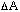is the average slope of indifference curve Ua between F and D. The slope of mL and Ln are almost equal, indicating that it does not matter whether you measure value in terms of a little more of a good or a little less, provided you consider only very small changes.

#### Preferences: The Shape of Indifference Curves

All of the indifference curves I have drawn have a similar shape--they slope down and to the right, and the slope becomes less steep the farther right you go (this shape is sometimes described as convex or convex to the origin). Why?

The curves slope down to the right because both apples and oranges are goods. If one bundle (J) has more of both apples and oranges than another (C), so that a line through them would slope up and to the right, both points cannot be on the same indifference curve. You would obviously prefer J, which has more of both goods, to C. But an indifference curve connects bundles among which you are indifferent. So if a bundle (C) has more apples than another on the same indifference curve (D), putting it farther right, it must have fewer oranges--putting it lower. So indifference curves must slope down to the right, up to the left. If, for some (large) quantity of apples, apples became a bad (you have so many that you would prefer fewer to more), the indifference curve would start to slope up; in order to keep you on the same indifference curve, additional apples (a bad) would have to be balanced by additional oranges (a good).

Two different indifference curves cannot intersect. If they did, the point of intersection would represent a bundle that was on both curves, and therefore had two different utilities. A different way of saying the same thing is to point out that if two indifference curves do intersect, they must have the same utility (the utility of the bundle that is in both of them), and are therefore really one indifference curve.

What can we say about the shape of the curve? As you move from point F to point D along Ua, the number of apples increases byand the number of oranges decreases by. Since F and D are on the same indifference curve (Ua), you are indifferent between them. That implies thatapples have the same value to you asoranges; one apple is worth/oranges./is the value of an apple measured in oranges. It is also (minus) the slope of the line FD--which is approximately equal to the slope of Ua between F and D (more nearly equal the smallerandare). The slope gets less steep as you move down and to the right along the indifference curve, because the value of apples measured in oranges becomes less as you have more apples (farther right) and fewer oranges (lower). This is the same pattern we already saw in Table 3-1.

Figure 3-1 also allows us to see geometrically why the meaning of the value of apples becomes less ambiguous the smaller the changes (in quantity of apples and oranges) we consider. Suppose we start at point L on indifference curve Uc . For large changes in both directions, the two ways of calculating the value of an apple (how many oranges would you have to get to make up for losing one apple versus how many oranges would you be willing to lose in exchange for getting one apple) correspond to finding the slopes of the lines LM and LN, which are substantially different. For small changes, they correspond to finding the slopes of the shorter lines Lm and Ln, which are almost equal. As the change approaches zero, the two slopes approach equality with each other and with the slope of the indifference curve at L.

The indifference curves on one figure in a textbook are usually very similar; sometimes they are simply the same curve shifted to different positions. In part that is because it is easier to draw them that way, in part because for many utility functions indifference curves that are close to each other have similar shapes. It need not, however, always be true.

Numerical Example

In Figure 3-1, point D is a bundle of 4 apples and 3 oranges, and point F is a bundle of 2 apples and 8 oranges.is 2 apples andis 5 oranges. The slope of the line connecting D and F is (minus) 5/2. 5/2 is also the value of an apple--2 apples are worth 5 oranges, so an apple is worth 5/2 of an orange.

#### Finding the Optimal Bundle

In the previous section, we considered an individual who started with a particular bundle of apples and oranges (A) and could trade apples for oranges at a rate of 1 for 1. In this section, we will analyze essentially the same situation, starting out in a slightly different way. We begin by assuming that you have an income (I), which you can use to buy apples and oranges; the price of apples is Pa and the price of oranges is Po. If you spend your entire income of \$100 on apples at \$0.50 apiece, you can buy I/Pa (\$100/\$0.50 = 200) apples and no oranges, putting you at point K on Figure 3-2a. If you spend your entire income on oranges at \$1 apiece, you can buy I/Po (\$100/\$1 = 100) oranges and no apples, putting you at point L. You should be able to convince yourself, by either algebra or trial and error, that the line B connecting L and K (called thebudget line) represents all of the different combinations of apples and oranges that you could buy, using your entire income. Its equation is I = a(Pa) + o(Po) where a is the quantity of apples you buy and o is the quantity of oranges. Put in words, that says that the amount you spend on apples and oranges equals quantity of apples times price of apples plus quantity of oranges times price of oranges--equals your entire income. Remember that at this point, apples and oranges are the only goods that exist.

Numerical Example

Suppose your income is \$100/month; Pa = \$0.50/apple; Po = \$1/orange. Table 3-2 shows some of the different bundles that you could buy with your \$100 income. Figure 3-2a shows the corresponding budget line.

Table 3-2

 Apples Oranges Expenditure 200 0 200 apples x \$0.50 + 0 oranges x \$1 = \$100 160 20 160 apples x \$0.50 + 20 oranges x \$1 = \$100 120 40 120 apples x \$0.50 + 40 oranges x \$1 = \$100 100 50 100 apples x \$0.50 + 50 oranges x \$1 = \$100 60 70 60 apples x \$0.50 + 70 oranges x \$1 = \$100 20 90 20 apples x \$0.50 + 90 oranges x \$1 = \$100 0 100 0 apples x \$0.50 + 100 oranges x \$1 = \$100

Indifference curves, such as those of Figure 3-1, show a consumer's preferences. The budget line plus the region below it (bundles that cost less than his income) show the alternatives available to him--his opportunity set. Figure 3-2a shows both.

The bundles on indifference curve U5 are preferred to those of the other two curves; unfortunately there is no point that is both on U5 and on (or below) the budget line--no bundle on U5 that the consumer can buy with his income. There are two points on U1 that are also on the budget line (M and N), representing two bundles that the consumer could buy; in addition, the portion of U1 between the two points is below the budget line and therefore represents bundles that the consumer could buy and still have some money left over. Should the consumer choose one such point? No. Points 0 and P are on both the budget line and U2; since U2 is above (hence preferred to) U1, the consumer prefers 0 (or P) to M or N or any other bundle on U1.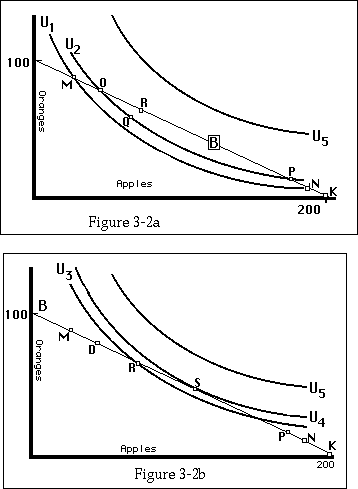The solution to the consumer-choice problem for a world of only 2 goods. B is the budget line for a consumer who has \$100 and can buy oranges at \$1 each or apples at \$0.50 each. The optimal bundle is S, where the budget line is tangent to an indifference curve, since there is no point on B that is on a higher indifference curve than U4.

Should the consumer choose a bundle represented by O, P, or one of the points in between, such as Q? Again the answer is no. Remember that the three indifference curves are merely the three I have chosen to draw out of the infinite number needed to describe the consumer's preferences. Consider point R. It represents a bundle containing more of both goods than Q; hence it is preferable to Q. Since all points on U2 are equivalent, R must also be superior to O and P. To find out whether it is the best possible bundle, we draw the indifference curve on which it lies--U3 on Figure 3-2b. As I have drawn it, there is another point, S, that lies on a still higher indifference curve and is also on the budget line.

Should the consumer choose S ? Yes. Its indifference curve, U4, just touches the budget line. Since any higher indifference curve must be above U4, it cannot intersect the budget line. S is the optimal bundle.

It appears that the highest indifference curve consistent with the consumer's income is the one that is just tangent to the budget line, and the optimal bundle is at the point of tangency. This is the usual solution; Figures 3-3a and 3-3b show two exceptions. In each case, the budget line is the same as in Figures 3-2 but the indifference curves are different; the figures represent the same income and prices as Figures 3-2 but different preferences.

On Figure 3-3a, the consumer's optimal point is X on indifference curve U2. He could move to a still higher indifference curve by moving down and to the right along the budget line--except that to do so, he would have to consume a negative quantity of oranges! Similarly, in Figure 3-2b, in order to do better than point Y, he would have to consume a negative quantity of apples. These are both corner solutions. In the normal case (interior solution), where the optimal bundle contains both apples and oranges, the result of the previous paragraph holds--the optimal bundle is at the point of tangency.Corner solutions on an indifference curve diagram. X shows a situation in which the consumer's preferred bundle contains only apples; Y shows a situation in which it contains only oranges.

#### Price = Value

If two lines are tangent, that means that they are touching and their slopes are the same. The budget line runs from the point (0,I/Po) to the point (I/Pa,0), so its slope is -(I/Po)/(I/Pa) = -Pa/Po. The rate at which you can trade apples for oranges (while keeping your total expenditure fixed) is simply the ratio of the price of an apple to the price of an orange. That is the same thing as the price of an apple measured in oranges; if apples cost \$0.50 and oranges \$1, then in order to get one more apple you must give up half an orange. The price of an apple (measured in oranges) is half an orange. So the slope of the budget line is minus the price of an apple measured in oranges.

The slope of the indifference curve, as I showed earlier in this chapter, is minus the value of an apple measured in oranges. So, in equilibrium, the price of an apple measured in oranges (the rate at which you can transform oranges into apples by selling one and buying the other) is equal to the value of an apple measured in oranges (the marginal rate of substitution--the rate at which oranges substitute for apples as consumption goods, the number of oranges you are willing to give up in exchange for an apple). This is the same result that I sketched verbally at the end of the first part of the chapter, when I said that you would keep trading until you reached a point where the value of an additional orange (in apples) was equal to its cost (also in apples).

One possible reaction to this result is "that's obvious; of course the value of something is the same as its price." Another is "this is a bunch of meaningless gobbledygook." Both are wrong.

To see why the first reaction is wrong, consider what we mean by price and value. Price is what you have to give up in order to get something. Value is what you are just barely willing to give up to get something. Nothing in those two concepts makes it obvious that they are the same.

The second reaction is much more defensible. You have just been bombarded with a considerable junkpile of abstractions; it may take a while to dig yourself out. You may find it useful to go through the argument in each of the four ways it is presented (two so far, two in the next chapter) until you find one that makes sense to your intuition. Once you have done that, you should be able to go back over the other three and make sense out of them too. One of the reasons for using several different languages is that different people learn in different ways.

This equality between relative prices and relative values is one example of a very general pattern that we will see again and again. I will refer to it as the equimarginal principle--marginal because the values being compared are values for one more apple, orange, or whatever. It is a statement not about our tastes but about equilibrium--where we are when we stop trading. The same pattern has already appeared several times, in a very different context, in the optional sections of Chapter 1, where we saw that in equilibrium all lines in a supermarket and all lanes on a freeway are equally attractive--provided that the cost of getting to them is the same.

#### The Invisible World--A Brief Digression

Another response you may have at this point is "Where do all these tables and indifference curves come from, anyway? How can you possibly know what my preferences are? How, for that matter, can I know exactly how many apples I would give for an orange? Are economists people who go around asking people what bundles they are indifferent among--and are they fools enough to believe the answers?"

I shall answer the five questions in order. The tables and figures all came out of my head--I made them up, subject to the requirement that the numbers in the table have a certain pattern and the curves a certain shape. I cannot tell what your preferences are. You do not know exactly what your preferences are. No. No.

If we cannot find out what indifference curves are, what good are they? The answer is that indifference curves--like much of the rest of economics--are tools used to help us think clearly about human behavior. By using them, we can show that if people have preferences and rationally pursue them (the assumptions that I made and defended in Chapter 1), certain consequences follow. So far in this chapter, I have concentrated on one particular consequence--the equality between relative values and relative prices. I will show others later. Indifference curves and the like are useful as analytical tools; it is a serious error to think of them as things we actually expect to go out and measure.

#### Income and Substitution Effects

Now that we know what indifference curves are, we shall use them to show how the amount you consume of a good varies with your income and with the price of the good. Figure 3-4 shows what happens as income rises, with price held constant. B1 is the same budget line we have seen before, corresponding to an income of \$100 and prices of \$1/orange and \$0.50/apple. B2 is the budget line for the same prices but for an income of \$125, B3 for an income of \$150. Since relative prices are the same in all three cases, all three budget lines have the same slope, making them parallel to each other. In each case, I have drawn in the indifference curve that is just tangent to the budget line. As income rises, the consumption bundle shifts from X to Y to Z; in the case illustrated, consumption of both apples and oranges rises with income--they are normal goods. The line IEP is the income ixpansion path showing how consumption of apples and oranges changes as the consumer's income increases.Optimal bundles for three different incomes--2 normal goods. X is the optimal bundle for an income of \$100, Y for an income of \$125, and Z for an income of \$150--as shown by B1, B2, and B3. Consumption of both apples and oranges increases with increasing income.

Figure 3-5 shows the same pattern of income and prices but a different set of indifference curves, corresponding to an individual with different preferences. This time, as income increases, the consumption of oranges increases but the consumption of apples decreases! In such a situation, apples are an inferior good--a good of which we consume less the richer we are. Hamburger and beans are both plausible examples of inferior goods, for some ranges of income. As a very poor person becomes less poor, he eats hamburger instead of beans; his consumption of beans goes down as his income goes up, so for that range of incomes beans are an inferior good. As his income becomes still higher, he starts eating steak instead of hamburger. His consumption of hamburger goes down as his income goes up, so for that range of incomes, hamburger is an inferior good.

In describing the budget lines B1, B2, and B3, I gave specific values for income and prices. I could just as easily have told you that income was \$200, \$250, and \$300 and that prices were \$2/orange and \$1/apple; that would have produced exactly the same budget lines. The reason is obvious: If you double your income and simultaneously double the price of everything you buy, your real situation is unchanged--you can buy exactly the same goods as before.

I could also have told you that income was \$100 for all three budget lines and that the price of an orange was \$1 for B1, \$0.80 for B2, and \$0.66 2/3 for B3, with corresponding prices (\$0.50, \$0.40, \$0.33 1/3) for apples. A drop in the price of everything you consume has the same effect on what you can buy as an increase in income.

It is not obvious when we should describe changes on an indifference curve diagram--or changes in the situations that such diagrams represent--as changes in prices and when we should describe them as changes in income. That is not because there is something wrong with indifference curves but because the distinction between a change in income and a change in price is less clear than it at first seems. We are used to thinking of prices and incomes in terms of money, but money is important only for what it can buy; if all prices go down and my income stays the same, my real income--my ability to buy things--has risen in exactly the same way as if prices had stayed the same and my money income had gone up.

If income and prices all change at once, how can we say whether my real income has gone up, gone down, or stayed the same? Income is useful for what it can buy; the value to me of the bundle of goods that I buy is indicated, on an indifference curve diagram, by what indifference curve it is on. It therefore seems natural to say that a change in money income and prices that leaves me on the same indifference curve as before has left my real income unchanged. A change that leaves me on a higher indifference curve has increased my real income; a change that leaves me on a lower indifference curve has lowered my real income.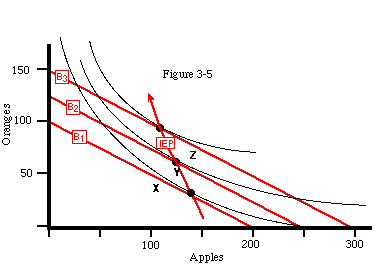Optimal bundles for three different incomes--a normal good and an inferior good. As income increases, consumption of oranges increases but consumption of apples decreases; so apples are an inferior good. IEP is the income expansion path.

The prices that are important are relative prices--how much of one good I must give up to get another. As I showed earlier, the price of one good in terms of another corresponds to (minus) the slope of the budget line. So a change in money income and money prices that alters the slope of the budget line while leaving me on the same indifference curve is a pure change in prices--prices have changed and (real) income has not. A change that leaves the slope of the budget line the same but shifts it so that it is tangent to a different indifference curve is a pure change in income--real income has changed but (relative) prices have not. An example of the former is shown on Figure 3-6a; an example of the latter, on Figure 3-6b.

Figure 3-7 shows the effect of a decrease in the price of apples. B1 is the same budget line as before; A is the optimal bundle on B1. B2 is a budget line for the same income (\$100) and the same price of oranges (\$1/orange), but for a new and lower price of apples (0.33 1/3/apple). C is the optimal point on that budget line. We can decompose the movement from point A to point C into two parts, as shown in Figure 3-7. A pure change in price with real income fixed would leave us on the same indifference curve, changing the budget line from B1 to B' and the optimal bundle from A to B. A pure change in income would keep relative prices (the slope of the budget line) unchanged, while moving us to a different indifference curve. That is the movement from bundle B on budget line B' to bundle C on budget line B2; note that B' and B2 are parallel to each other. The change in our consumption as we move from A to B is called the substitution effect (we substitute apples for oranges because they have become relatively cheaper); the change as we move from B to C is called the income effect.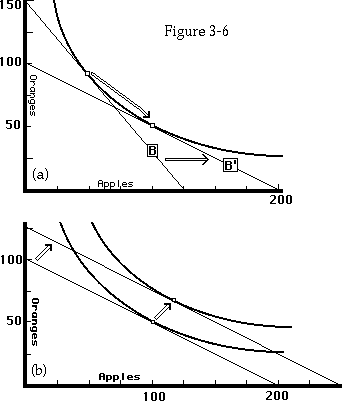A pure change in price (a) and a pure change in income (b). On Figure 3-6a, relative prices change, but real income does not, since the individual ends up on the same indifference curve after the change. On Figure 3-6b, relative prices stay the same but real income increases.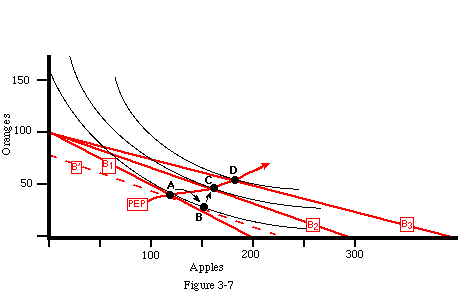The effect of a fall in the price of apples. When the price of apples falls, the optimal bundle changes from A to C. The movement from A to B is a substitution effect--relative prices change, real income does not. The movement from B to C is an income effect; real income changes, relative prices do not. A further price drop moves the optimal bundle to D. The line PEP, running from A to C to D, is the price expansion path.

A pure substitution effect always increases the consumption of the good that has become relatively cheaper. You can see that by looking at the shape of the indifference curve and imagining what happens as the budget line "rolls along it" (as it does from B1 to B' ). This corresponds to lowering the price of one good while at the same time cancelling out the gain to the consumer by either raising the price of the other good or lowering income. On net, the consumer is neither better off nor worse off. The result is to increase the consumption of the good that has become cheaper. The pure income effect from a decrease in the price of a good (an increase in real income), on the other hand, may either increase or decrease its consumption, according to whether it is a normal or an inferior good.

A drop in the price of one good without any compensating change in income or other prices produces both a substitution effect and an income effect, as shown on Figure 3-7; apples are cheaper than before relative to oranges, and the lower price of apples makes the consumer better off than before. The substitution effect always increases the consumption of the good whose price has fallen; the income effect may increase or decrease it. You can see the net effect by looking at the price expansion path (PEP on Figure 3-7), which shows how consumption of both goods changes (from A to C to D ) with changes in the price of one good.

This suggests the possibility of a good so strongly inferior that the income effect more than cancels the substitution effect--as its price falls, its consumption goes down. Imagine, for example, that you are spending most of your income on hamburger. If the price of hamburger falls by 50 percent while your income and all other prices remain the same, your real income has almost doubled. Since you are now much richer than before, you may decide to buy some steak and reduce your consumption of hamburger. The substitution effect tends to make you consume more hamburger; at the lower price of hamburger, the money required to buy an ounce of steak would buy twice as much hamburger as before the price change; so steak is more expensive in terms of hamburger than before. But you are now much richer--so you may choose to eat more steak in spite of its higher relative cost.

A good whose consumption goes down instead of up when its price goes down is called a Giffen good. It is not clear whether any such goods actually exist. The reason is that most of us consume many different goods, spending only a small part of our income on any one. A drop in the price of one good has a large effect on its relative price (hence a large substitution effect) but only a small effect on our real income. A Giffen good must either consume a large fraction of income or be so strongly inferior that the effect of a small change in income outweighs that of a large change in relative price.

Students frequently confuse the idea of an inferior good with the idea of a Giffen good. An inferior good is a good that you buy less of when your income goes up. There are many examples--for some of you, McDonald's hamburgers or bicycles. A Giffen good is a good that you buy less of when its price goes down. A Giffen good must be an inferior good, but most inferior goods are not Giffen goods.

If Giffen goods are rare or nonexistent, why have I spent time discussing them? The main reason is that in much of economic analysis (including a good deal of this book), we assume that demand curves slope down--that the higher the price of something is, the less of it you buy. If I am going to use that assumption over and over again, it is only fair to give you some idea of how solid it is--by describing the circumstances in which it would be false.

#### Demand Curves

Figure 3-6a showed the effect on consumption of a pure change in price. Figures 3-8a and b and Table 3-3 show how the same analysis can be used to derive an income-compensated demand curve (also known as a Hicksian demand curve after economist John Hicks). The budget lines on Figure 3-8a correspond to a series of different prices for apples, from \$0.50/apple to \$2/apple. The price of oranges is held constant at \$1/orange. Table 3-3 shows prices, quantities, and income for each budget line. Figure 3-8b is the resulting demand curve. It shows the relation between price of apples and quantity purchased for the consumer whose preferences are represented on Figure 3-8a. It is an income-compensated demand curve because, as we increase the price of apples, we also increase income by just enough to keep the consumer on the same indifference curve. We thus eliminate the income effect; the change in the quantity purchased is due to the substitution effect alone.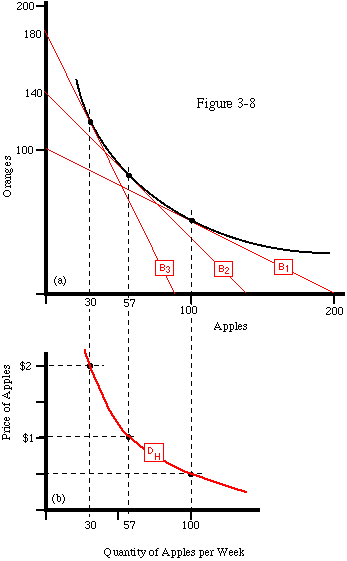The derivation of an income-adjusted demand curve. Budget lines B1, B2 and B3 show different combinations of prices and income corresponding to the same real income. DH is the resulting income-adjusted (Hicksian) demand curve.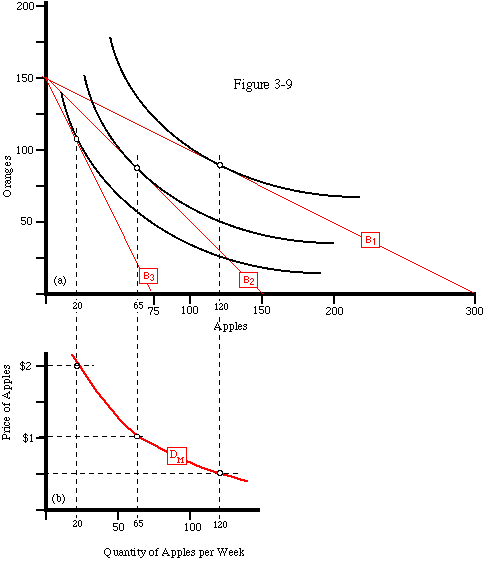The derivation of an ordinary demand curve. Budget lines B1, B2 and B3 show different prices of apples but the same income and price of oranges. DM is the ordinary (Marshallian) demand curve.

Figures 3-9a and b and Table 3-4 show the similar derivation of an ordinary demand curve (called a Marshallian demand curve after economist Alfred Marshall). This time, just as on Figure 3-7, the price of apples is changed while both the price of oranges and income are held constant. The higher the price of apples, the worse off the consumer; his dollar income is the same but, since his dollars will buy fewer apples, his real income is lower. So the higher the price of an apple on Figure 3-9a, the lower the indifference curve to which the corresponding budget line is tangent. This time the change in quantity purchased includes both an income and a substitution effect.

Table 3-3

 Budget Line Price of Apples Price of Oranges Income (\$/week) Quantity of Apples Purchased per Week B1 \$0.50 \$1.00 100 100 B2 \$1.00 \$1.00 140 57 B3 \$2.00 \$1.00 180 30

Table 3-4

 Budget Line Price of Apples Price of Oranges Income (\$/week) Quantity of Apples Purchased per Week B1 \$0.50 \$1.00 150 120 B2 \$1.00 \$1.00 150 65 B3 \$2.00 \$1.00 150 20

For most economic problems, the relevant demand curve is the Marshallian one, since there is generally no reason to expect a change in the price of one good to cause a compensating change in income or other prices. Some parts of economic theory however, including consumer surplus, which will be explained in Chapter 4, can be derived rigorously only by using income-compensated demand curves.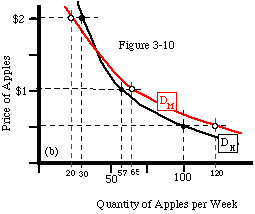Ordinary and income-adjusted demand curves for the same individual. DM is the ordinary (Marshallian) demand curve; DH is the income-adjusted (Hicksian) demand curve.

The Marshallian demand curve DM on Figure 3-9b and the Hicksian demand curve DH on Figure 3-8b are significantly different, as you can see on Figure 3-10. That is because we are considering a world with only two goods. Since raising the price of one of them makes the consumer significantly worse off, his behavior (the amount of the good he buys) is substantially different according to whether we do or do not compensate him for the change.

But in the real world, as I pointed out earlier, we divide our expenditure among many goods. If I spend only a small fraction of my income on a particular good, a change in its price has only a small effect on my real income. In such a case, the difference between the two demand curves is likely to be very small. For this reason, we will generally ignore the distinction between ordinary and income-compensated demand curves in what follows.

You have just bought a house. A month after you have concluded the deal, the price of houses goes up. Are you better off (your house is worth more) or worse off (prices are higher) as a result of the price change? Most people will reply that you are better off; you own a house and houses are now more valuable.

You have just bought a house. A month after you have concluded the deal, the price of houses goes down. Are you worse off (your house is worth less) or better off (prices are lower)? Most people, in my experience, reply that you are worse off. The answers seem consistent, even to those who are not sure what the right answer is. It appears obvious that if a rise in the price of housing makes you better off, then a fall must make you worse off, and if a rise makes you worse off, then a fall must make you better off.

Although it appears obvious, it is wrong. The correct answer is that either a rise or a fall in the price of housing makes you better off!

Before proving this, I will first describe the situation a little more precisely. I am assuming that you have an income (I), part of which went to buy the house. One may imagine either that your income is from a portfolio of stocks and bonds, part of which you sold in order to buy the house, or that you have a salary, part of which must now go for interest on the mortgage. In either case, you have bought housing and, as a result, have less to spend on other goods.

I am also assuming that none of the circumstances determining how much housing you want are ever going to change, except for the price of housing; if the price of housing stayed the same, so would the amount of housing you want. You are not, in other words, planning to have children and move to a bigger house or planning to retire, sell your house, and move to Florida. To simplify the argument, I will ignore all costs of buying, selling, or owning housing other than the price--sales taxes, realtor's commissions, and the like. Finally, I will assume that the change in price was unexpected; when you bought the house you were assuming that the price of housing, like everything else, was going to stay the same forever.

Now that I have described the situation more precisely, you may want to stop and try to figure out how my answer--that a change in either direction benefits you--can be true.

The situation is shown in Figure 3-11. The vertical axis represents housing; the horizontal axis represents expenditure on all other goods. The budget line B1 shows the different combinations of quantity of housing and quantity of other expenditure you could have chosen at the initial price of housing (\$50/square foot). Point A1 is the optimal bundle--the amount of housing you bought. It is on indifference curve U1.

Line B2 shows the situation after the price of housing has risen to \$75/square foot. B2, your new budget line, must have a slope of (minus) 1 square foot of housing/\$75, since that is the new price of housing--the rate at which you can exchange dollars spent on all other goods for housing, or vice versa. The new budget line must go through point A1, since one of the alternatives available to you is to do nothing--to keep the bundle that you had before the price change. You can choose to move away from point A1 along the budget line either up (buy more housing, trading dollars for housing at a rate of \$75/square foot) or down (sell your house and move to a smaller one--sell some of your housing for money at \$75/square foot). So your new budget line, B2, is simply a line with slope - 1/75 drawn through point A1.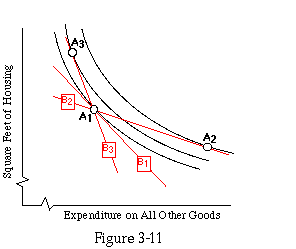The effect on a homeowner of a change in the price of housing. B1 shows the alternatives available at the original price of housing; B2 shows those available if the price of housing rises after the house is bought; B3 shows the alternatives available if the price falls. A1 shows the homeowner's bundle of housing and all other consumption after the house is built and before there is any change in housing prices. The change in the slope of the budget line has been exaggerated to make the effect clearer.

The figure shows what you choose to do; your new optimal point is at A2. Since housing is now more expensive than before, you have chosen to sell your house and buy a smaller one--the gain in income is worth more to you than the reduction in the amount of housing you consume. You are now on indifference curve U2, which is above (preferred to) U1.

Line B3 shows the situation if the price of housing goes down rather than up after you buy your house--to \$30/square foot. It is drawn in exactly the same way except that the price ratio is now 1/30. Again you have the choice of keeping your original house, so the line has to go through A1. Your new optimal point is A3; you have adjusted to the lower price of housing by selling your house and buying a bigger one. You are now on U3--which is above U1! The drop in the price of your house has made you better off.

By looking at the figures, you should be able to convince yourself that the result is a general one; whether housing prices go up or down after you buy your house, you are better off than if they had stayed the same. The same argument can be put in words as follows:

What matters to you is what you consume--how much housing and how much of everything else. Before the price change, the bundle you had chosen--your house plus whatever you were buying with the rest of your income--was the best bundle of those available to you. If prices had not changed, you would have continued to consume that bundle. After prices change, you can still choose to consume the same bundle. The house belongs to you, so as long as you choose to keep it, the amount of money you have to spend on other things is unaffected by the price of the house.

You cannot be worse off as a result of the price change--at worst you continue to consume the same bundle (of housing and other goods) as before. But since the optimal combination of housing and other goods depends, among other things, on the price of housing, it is unlikely that the old bundle is still optimal. If it is not, that means there is now some more attractive alternative, so you are now better off; a new alternative exists that you prefer to the best alternative (the old bundle) that you had before.

This seemingly paradoxical result is interesting in part for what it shows us about the relative virtues of our different languages. In solving the problem geometrically, the drawing tells us the answer. All we have to do is look at Figure 3-11 in order to see that any budget line that goes through A1 with a different slope than B1 has to intersect some indifference curve higher than U1--whether the slope is steeper (lower price of housing) or shallower (higher price of housing). What the drawing does not tell us is why it is true. When we solve the problem verbally, we are likely to get the wrong answer (as at the beginning of this section, where I concluded that a fall in the price should make you worse off). But once we do get the right answer (possibly with some help from the figures), we not only know what is true, we also understand why.

I have ignored the transaction costs associated with buying and selling houses--realtor's commissions, sales taxes, the time spent finding a satisfactory house, and so on. If such costs are included, the result is that small changes in housing prices have no effect at all on you--it is not worth paying the transaction costs necessary to increase or decrease your consumption of housing. Large changes in either direction benefit you.

#### Application: Subsidies

Figure 3-12 shows your preferences between potatoes and expenditure on all other goods. You have an income of \$150/week; the price of potatoes is \$3/pound. If you spend all your income on potatoes, you can consume 50 pounds per week of potatoes and nothing else. If you spend nothing on potatoes, you have \$150/week left to spend on all other goods. Line B is your budget line; point D is the bundle you choose.

The potato lobby convinces the government that potatoes are good for you and should therefore be subsidized. For every \$3 you spend on potatoes, the government gives you \$1. So for each pound of potatoes you buy, you have \$2 less (instead of \$3 less) to spend on other goods--the cost of potatoes to you is now only \$2/pound instead of \$3/pound.

If you choose to buy no potatoes, you are unaffected by the subsidy and can spend your entire income of \$150/week on other goods. If you choose to spend your entire income on potatoes, you can now buy 75 pounds per week. B1 is your new budget line. Your optimal bundle is D1. Your consumption of potatoes has risen. Since you are on a higher indifference curve than before--U4 instead of U2--you are better off than before. You are happier (and, if the potato farmers are right, healthier); the potato farmers are selling more potatoes; all is well with the world.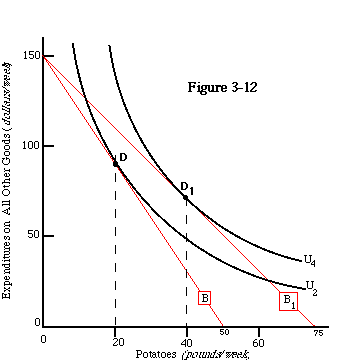The effect of a potato subsidy that someone else pays for. B is the initial budget line, B1 is the budget line after the government announces that it will pay one third of the cost of the potatoes you buy.

There is one problem. At point D1 you are consuming 40 pounds per week of potatoes (if that seems unreasonable, you may assume that some of the potatoes are converted into vodka before you consume them). Each pound costs \$3, of which you pay only \$2; the other dollar is provided by the subsidy. So the total subsidy is \$40/week. Some taxpayers somewhere are paying for that subsidy. Before we conclude that the potato subsidy is a complete success, we should include their costs in our calculations.

To do so, I will assume that consumers and taxpayers are the same people. For simplicity I will also assume that everyone has the same income and the same preferences, as shown on Figures 3-12 and 3-13. Each individual has a pretax income of I = \$150/week and an aftertax income of I - T, where T is the amount of tax paid. To find T on the figure, note that a consumer who buys no potatoes has I-T (income minus tax) available to spend on everything else, so I-T is the vertical intercept of the budget line. While we do not yet know what T is, we do know that the total amount collected in taxes must be the same as the amount paid out in subsidy (we ignore the cost of collecting taxes and administering the subsidy).

For the population as a whole, tax collected equals subsidy paid, and the amount of subsidy paid depends on how many pounds of potatoes people buy. But from the standpoint of each individual in a large population, the quantity of potatoes he buys has a negligible effect on the total subsidy and hence on his taxes. So each individual takes T as given and finds his optimal bundle, as shown on Figure 3-13. Since the effective price of potatoes is still \$2/pound (pay \$3 and get \$1 back as subsidy), the corresponding budget line (B') has the same slope as B1 on Figure 3-12.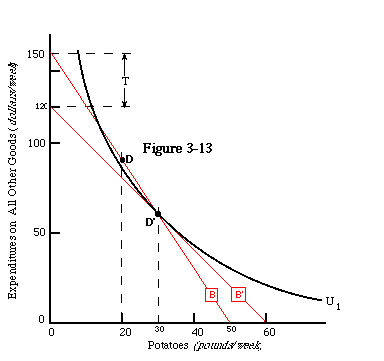The effect of a potato subsidy that you pay for. T is the tax that pays for the subsidy; B' is the budget line for a consumer who pays the tax and can receive the subsidy. At D', the optimal point on B', the consumer pays exactly as much in tax as he receives in subsidy&endash;and is wose off than he would be, at D, if neither tax nor subsidy existed.

How do we know that the budget line is B', instead of some other line with the same slope? B' is the only budget line for which the tax collected from each taxpayer (T = I-(I-T)=\$150/week-\$120/week=\$30/week) is exactly equal to the subsidy paid to each taxpayer (\$1/lb x 30 lb/week at point D')--as it must be, since everyone is identical and total taxes paid must equal total subsidy received.

When Is a Wash Not a Wash? D', the bundle you choose to consume, lies on both B', your budget line given the tax and subsidy, and B, your original budget line. This is not an accident. In the simple case I have described, everyone buys the same amount of potatoes, receives the same amount of subsidy, and pays the same amount of taxes; so taxes and subsidy must be equal not only for the population as a whole but for each individual separately. If you pay as much in taxes as you receive in subsidy, tax and subsidy cancel; the bundle (potatoes plus expenditure on all other goods) that you purchase is one you could have purchased from your original income if there had been neither tax nor subsidy. So it must be on B, your initial budget line.

That, in fact, is how I found B' in the first place. I knew that B' had to be parallel to B1. I also knew that its optimal point, where it was tangent to an indifference curve, had to occur where it intersected B. B' was the only line that met both conditions.

D' is on a lower indifference curve than D--the combination of tax and subsidy makes you worse off. This is not accidental. Since D' is on your original budget line, it is a bundle that you could have chosen to consume if there had been no subsidy and no tax. In that situation, you chose D instead, so you must prefer D to D'. So the combination of a subsidy and a tax that just pays for the subsidy must make you worse off.

In accounting, a transaction that results in two terms that just cancel--a \$1,000 gain balanced by a \$1,000 loss--is referred to as a wash. Your first reaction on reading the previous few paragraphs may be that the sort of tax/subsidy combination I have described is a wash; since you are getting back just as much as you are paying, there is no net effect at all.

In one sense, that is true; in another and more important sense, it is not. The total dollar value of your consumption bundle is the same with or without the tax/subsidy combination; in that sense, there is no effect. But, as you can see on Figure 3-13, the bundle you choose is different in the two cases; with the tax and subsidy, you end up choosing a less attractive consumption bundle--one on a lower indifference curve--than without it.

The reason for the difference goes back to a point I made earlier--that although the amount of the tax is determined for the population as a whole by how many pounds of potatoes are consumed, each individual will and should treat the amount of the tax as a given when deciding how many potatoes to buy. Given what everyone else is doing, your budget line (with the tax and subsidy) is B', not B. Since B' does not include D, you do not have the option of choosing that bundle. All of us, acting together, could choose D; each of us, rationally responding to the subsidy and the rational behavior of everyone else, chooses D'. This seemingly paradoxical result--that in some situations, rational behavior by every individual leaves each individual worse off--is not new. We encountered it before when we were explaining why armies run away and traffic jams.

Where You Are Going, Not How You Get There. Students faced with something like the potato subsidy problem often make the mistake of trying to solve it in stages. First they draw the budget line representing the subsidy (B1). From that they calculate how many potatoes the consumer buys, then from that they calculate the amount of the tax necessary to pay for the subsidy. The problem with this approach is that imposing the tax shifts the budget line, which changes the number of potatoes consumed, which changes the amount of subsidy paid out, which changes the amount of tax needed to pay for the subsidy! You are caught in an infinite loop; each time you solve one part of the problem another part changes.

The solution is to ignore the series of successive approximations by which someone trying to find the tax that just paid for the subsidy would grope his way towards the solution, and simply ask what the solution must look like when he has finally reached it. That is what we did on Figure 3-13. A subsidy of \$1/lb implies a budget line parallel to B1. A tax that just pays for the subsidy implies a budget line whose optimal point (where it is tangent to an indifference curve) occurs where it intersects B--meaning that consumers with that budget line buy a quantity of potatoes such that the tax just pays for the subsidy. B' is the only budget line you can draw on Figure 3-13 that meets both of those conditions, so it must be the solution.

Fine Point. One assumption implicit throughout this discussion is that the tax/subsidy does not affect the market price of potatoes; that was always assumed to be \$3/pound. The assumption is a reasonable one if we imagine that the subsidy and tax apply to only a small part of the population--say, a single town. Changes in the potato consumption of Podunk are unlikely to have much effect on the world market price of potatoes. It is less reasonable if we consider a program applying to the entire population of the United States. One effect of the subsidy is to increase the demand for potatoes, which should produce an increase in their price. That is one of the reasons why the potato farmers are in favor of the subsidy.

This raises a second question. So far, in analyzing the problem, we have only considered the interests of the consumers and the taxpayers; what about the producers? Is it possible that if we take them into account as well, the net effect of the subsidy is positive?

Insofar as we can answer that question--insofar, in other words, as we have a way of adding up different people's gains and losses--the answer is no. Even including the effect on the producers, the net effect of the subsidy is negative. You will have to wait until Chapter 17 to learn why.

#### Other Constraints

The same techniques that we have been using to analyze the constraint imposed upon a consumer by his limited income could just as easily be used to analyze other sorts of constraints. Consider, for example, someone on a thousand calorie/day diet. He faces a calorie constraint. Each food has a price in calories per ounce; he must choose a bundle of foods whose total cost is no more than a thousand calories. If he is considering only two alternative foods the thousand calorie bundles will lie along a budget line; his optimal bundle will be where that budget line is tangent to an indifference curve.

There is another constraint that applies to everyone, even those fortunate enough not to have to diet. Most things we do, including earning money and spending it, require time. Each of us must allocate his limited budget of 24 hours a day among a variety of uses--work, play, consumption, rest. If we consider only two alternatives, holding the rest fixed, we again have a choice that can be represented by an indifference curve diagram.

OPTIONAL SECTION

UTILITY FUNCTIONS

#### Utility

Utility and the utility function were important ideas in the development of economics and remain useful as tools for thinking about rational behavior. The idea of utility grows out of the attempt to understand all of an individual's choices in terms of a single thing he is trying to maximize--happiness, pleasure, or something similar. We call this his utility. Utility is observed only in choices. The statement "The utility to you of a Hawaiian vacation is greater than the utility to you of a moped" is equivalent to the statement "Given the choice between a Hawaiian vacation and a moped, you would choose the vacation." It does not mean "A vacation is more useful to you than a moped." Used as a technical term in economics, utility does not have the same meaning as in other contexts.

A utility function is a way of describing your preferences among different bundles of goods. Suppose we consider only two goods--apples and pears. The statement "Your utility function is 3 x (number of pounds of apples) + 2 x (number of pounds of pears)," which we write mathematically as

u(a,p) = 3a + 2p,

means that if you have to choose between two bundles of apples and pears, you will choose the bundle for which that function is greater. You will prefer four pounds of apples plus three pounds of pears (total utility = 18) to three pounds of apples plus four pounds of pears (total utility = 17).

If you are not familiar with functions, you may find the expression u(a,p) confusing. All it means is "utility, which depends on a (the number of pounds of apples) and p (the number of pounds of pears)." The form of the dependence is then shown on the other side of the equality sign.

Several things are worth noting about such functions. The first is that we are very unlikely to know what someone's utility function actually is--we would have to know his preferences among all possible bundles of goods. The purpose of utility functions is to clarify our thinking by allowing us to build simplified pictures of how people act. Such models are not attempts to describe reality; they are attempts to set up a simplified situation with the same logical structure as the much more complicated reality in order to use the former to understand the latter. You should not confuse such models with large-scale econometric models--complicated sets of equations used (not very successfully) to try to predict the behavior of some real-world economy.

The second point to note is that the same pattern of behavior can be described by many different utility functions. In the example given above, suppose the utility function had been not u but

v(a,p) = 6a + 4p = 2 x u(a,p).

The second function (v) is just twice the first (u); if the first is larger for one combination of apples and oranges than for another, so is the second. An individual always chooses the bundle that has higher utility, so the two utility functions imply exactly the same behavior.

So far, we have assumed that your utility depends on only two goods. More generally we can write u(x), where x is a bundle of goods. In the simple two-good case, x is the number of apples and of pears; we could write x = (2,3) to describe a bundle of 2 apples and 3 pears. In the more general case of n goods, x is a longer list, describing how much of each good is in the particular bundle being considered. If we call the first good X1 and the amount of the first good x1, the second good X2 and the amount of the second good x2, and so on, and if the price of the first good is P1 and similarly for the other goods, your income constraint--the requirement that the total bundle you purchase is worth no more than your total income--is the equation

I >= P1x1 + P2x2 + . . . + Pnxn,

where the right-hand side of the equation is the amount you have to spend to buy that quantity of the first good (the quantity times its price--3 pounds of apples at \$1/pound equals \$3), plus the amount for the quantity you are buying of the second good, plus ... .

The point I made above about equivalent utility functions can be made more general by observing that if there are two functions, u(x), v(x), and if for any two bundles of goods, xa,xb, whenever u(xa) > u(xb) then v(xa) > v(xb) and vice versa, then the two utility functions describe exactly the same behavior and are equivalent. The purpose of a utility function is to tell which bundle of goods I prefer (the one for which the utility function gives a higher utility). Two different functions that always give the same answer to that question are equivalent--they imply exactly the same preferences.

My income and the prices of the goods I want define the alternatives from which I can choose; my utility function defines my preferences. Mathematically speaking, the problem of consumption is simply the problem of choosing the bundle of goods that maximizes your utility, subject to the income constraint--the requirement that the bundle you choose cost no more than your income. This, of course, is what we were doing earlier in the chapter. The utility function simply provides a more mathematically precise way of talking about it.

#### Calculus

We have a utility function u(x,y,z, . . .) depending on the amount consumed of goods X, Y, Z, etc. We assume that the quantities x, y, z, . . . can be continuously varied; that for x, y, z, . . . > 0, u is a continuous function with continuous first derivatives, and that u is an increasing function of all its arguments (since they are goods, utility increases with increased consumption). u obeys the principle of declining marginal utility: du/dx decreases as x increases (and similarly for y, z, etc.), so [[partialdiff]] 2u/[[partialdiff]] x2 < 0 . Our problem is to maximize u subject to the income constraint:

I > xPx + yPy + zPz + . . .

The general approach to solving such a problem (a constrained maximization) uses a mathematical device called a Lagrange multiplier, with which you may already be familiar. In this particular case, we can use a simpler and (to me) more intuitive approach. To begin with, note that > in the income constraint can be replaced by = ; since the only thing money is good for is buying goods and since more goods are always preferred to fewer goods, there is never any reason to spend less than your entire income.

We now consider varying x and y, while holding fixed the quantities of all other goods. If utility is at a maximum, an infinitesimal increase in x combined (because of the income constraint) with an infinitesimal decrease in y (such that total expenditure on x and y is unchanged) must leave utility unchanged, or in other words:

0=du(x,y,z, ...)=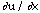dx +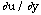dy

From which it follows that:

0 = du(x,y,z, . . .)/dx = [+dy/dx (Eqn. 1)

To find dy/dx we solve the income constraint for y in terms of x then take the derivative, thus:

y = (I - zPz - . . . - xPx)/Py

dy/dx = -(Px/Py).

Substituting this into Equation 1, we have

0 =- (Px/Py)Rearranging this gives us/Px =/Py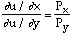which is the same relation that we derived earlier in the chapter, when we concluded that the price of an apple measured in oranges (Pa/Po) is equal to the value of an apple measured in oranges.is the marginal utility of x andthe marginal utility of y; their ratio is the value of x measured in y--the marginal rate of substitution. If a pound of X has a marginal utility of 3 and a pound of Y has a marginal utility of 1, then on the margin a pound of X is worth 3 pounds of Y. We could have made the same argument for X and Z instead of X and Y, or for any other pair of goods (holding consumption of everything else constant), so the equimarginal principle holds for all goods we consume.

It does not hold for goods we do not consume. As you may remember from a calculus course, the normal condition for a maximum, which is that the derivative is zero, does not apply if the maximum occurs at one end or the other of the variable's range. The situation is shown in Figure 3-14; f(x), which is only defined for x > 0, has its maximum value at x = 0. Its derivative there is negative, but we cannot find higher values of f at lower values of x because there are no lower values of x. This is a corner solution; the maximum occurs at the corner (point A on Figure 3-11), where the function runs into the barrier at x = 0.A corner solution. At A, f(x) is maximum, but df/dx is not zero.

A corner solution arises in consumption if there is a good X such that your maximum utility occurs when you are consuming none of it: x = 0. Since it is a corner solution, the derivative of utility need not be zero even though utility is at a maximum; so Equation 1 need not hold. Put in words, that means that utility is still increasing as you decrease consumption of the good (and spend the money on other goods) up to the point where your consumption of X reaches 0. The marginal utility of X is less per dollar than the marginal utility of other goods, but you cannot increase your utility by consuming a dollar less of X and a dollar more of something else since you have already reduced your consumption of X to zero. So the equimarginal principle does not apply to goods you do not consume. This is the same point I made earlier and illustrated on Figures 3-3a and 3-3b. The picture of the corner is different, since it involves a utility function here and an indifference curve there, but the situation is the same.

#### Indifference Curves and Utility Functions

Next let us look at indifference curve analysis in terms of the utility function. Since we have only two dimensions, we will limit ourselves to a utility function with only two goods. Even in that case, showing two goods uses up the two dimensions we have available, leaving no place to show the utility function itself With a third dimension, we could draw it as a surface, letting the height of the surface above any point (x,y) represent u(x,y). Unfortunately this book is written on two-dimensional paper; Figure 3-15a is an attempt to overcome that limitation.

This is not a new problem; mapmakers face it whenever they try to represent a three-dimensional landscape on two-dimensional paper. The solution is a contour map. A contour map has one line through all points 100 feet above sea level, another through all points 200 feet above, and so on; by looking at the map you can, with practice, figure out the shape of the land in the third dimension. Where it is rising steeply, the contours are close together (the land rises 100 feet in only a short horizontal distance); where it is gently sloped, they are far apart.

The economist's equivalent of the contour on a topographical map is an indifference curve; it represents all of the points among which you are indifferent--or in other words, all of the bundles that give you the same utility. Figure 3-15a shows indifference curves U1, U2, and U3, each labeled by its utility. Since Ul is less than U2, which in turn is less than U3, points on U3 are preferred to points on U2, which are in turn preferred to points on U1 . The X-Y plane of Figure 3-15a corresponds to the indifference curve diagrams done earlier in the chapter.

Indifference curves do not completely describe the utility function from which they come. A curve is not labeled; it does not say "utility equals 9" on it. All the indifference curves tell us is which bundles we are indifferent among and which we prefer to which. They are in this sense less informative than the lines on a contour map, which tell us not only where the contour is but which contour (how many feet above sea level) it is. Hence one set of indifference curves may correspond to many different utility functions. The fact that, in spite of that, we can analyze consumption completely in terms of indifference curves corresponds to a point I made earlier--that different utility functions may describe exactly the same behavior.

[I have omitted Figure 3-15 from the webbed version of this chapter; my publisher holds copyright on the figures that he had drawn and I'm not up to doing 3-D perspective. I will try to get permission to scan it in Real Soon Now. Use your imagination.]

As we move along an indifference curve, utility stays the same. Suppose (x,y) and (x + dx,y + dy) are two points on the same indifference curve. We have:

u(x,y) = u(x + dx,y + dy)u(x,y) + dx+ dyAs dy, dx --> 0, their ratio becomes the slope of the indifference curve, and the approximate equality becomes an equality. In that case,

dx+ dy= 0, and

-()/= dy/dx = slope of the indifference curve.

This is equivalent, as you should be able to show, to the conclusion we reached earlier--that minus the slope of the indifference curve was equal to the value of apples (X) measured in oranges (Y).

I1 in Figure 3-15b, like the indifference curves discussed earlier, slopes down to the right; its slope is negative. To keep utility constant, a reduction in the amount of one good must be balanced by an increase in the amount of another. Indifference curves sloping the other way would describe your preferences between two things, one of which is a good and one a bad--something for which> 0. If this seems an odd thing to graph, consider representing your utility as a function of number of hours worked and number of dollars of income, the first a bad and the second a good, and deducing how many hours you will work at any given wage. Or consider the situation where production of a good results in undesirable waste products.

The slope of an indifference curve is usually negative because we are usually representing preferences between two goods. Its curvature, the fact that the slope of the indifference curves becomes shallower (i.e., less negative) as you move right or down on the diagram and steeper as you move left or up, is suggested by the principle of declining marginal utility but is not, strictly speaking, implied by it. Imagine that you move from A to B on Figure 3-15b. Quantity of Y stays the same and quantity of X increases, so [[partialdiff]] u/[[partialdiff]] x must decrease. The slope of the indifference curve is -([[partialdiff]] u/[[partialdiff]] x)/([[partialdiff]] u/[[partialdiff]] y), so the slope of the indifference curve through B is shallower than the slope of the indifference curve through A--unless [[partialdiff]] u/[[partialdiff]] y decreases even faster than [[partialdiff]] u/[[partialdiff]] x as x increases. There is no obvious reason why it should, but nothing in our assumptions makes it impossible. Similarly, as you move from C to D, y increases, x stays the same, [[partialdiff]] u/[[partialdiff]] y decreases, and the slope of the indifference curves becomes steeper--unless, for some reason, an increase in the quantity of Y decreases the marginal utility of X even faster than it decreases the marginal utility of Y.

Here again, as several times before, our analysis is complicated by the possibility that consumption of one good may affect the utility of another. In most real-world situations, we would not expect such effects to be very large--we consume many different goods, most of which have little to do with each other. The exceptions are pairs of closely related goods--cars and bicycles, bread and butter, bananas and peanut butter. In some of these cases (substitutes, such as cars and bicycles) the more we have of one good the less we value the other; in other cases (complements, such as bread and butter or gasoline and automobiles) the more we have of one the more we value the other.

In such cases, we may expect indifference curves to be oddly shaped--some examples are given in the problems at the end of this chapter. In most other cases, we assume the principle of declining marginal rate of substitution--which means that the slope of the indifference curves becomes shallower as we move to the right on the diagram and steeper as we move up. As you can see from the previous discussion, this is close enough to the principle of declining marginal utility that for most practical purposes we may think of them as the same.

We have now derived the equimarginal principle directly from utility functions and shown the connection between utility functions and indifference curves. It is worth noting that although the argument was made in terms of money income and money prices, money has nothing essential to do with it. We could just as easily have started with a bundle of goods (x , y , . . .), and allowed you to exchange X for Y at a price (of Y in terms of X) of Py/Px, for Z at a price of Pz/Px, and so on. Here, as elsewhere in price theory, the use of money and money prices simplifies exposition but does not affect the conclusions.

#### PROBLEMS

1. Near the beginning of the chapter, I gave some examples of bads. Do you agree with them? If not, is one of us necessarily wrong? Discuss.

2. Suppose my preferences with regard to hamburger and pens are as shown.

 Options Hamburgers (pounds/year) Pens/year Utility (utiles/year) A 100 30 50 B 108 29 50 C 118 28 50 D 200 30 75 E ? 29 75

a. What is the value of a pound of hamburger to me (between points A and B )?

b. In choosing between bundles A and B, which do I prefer? Between C and D ?

c. About how much hamburger should be in E to make me indifferent between it and D ? Explain briefly.

3. Figure 3-16 shows your preferences between brandy and champagne. Which (if any) of the bundles shown do you prefer to point A ? To which is A preferred? Which are equivalent to A ? For which bundles can you not tell whether they are equivalent, better, or worse than A ?

4. Answer the same questions for point B.

5. Figure 3-17 shows your indifference curves for cookies and bananas. You have an income of \$100, the price of cookies is \$1, and the price of bananas is \$0.25. How many of each do you choose to consume?

6. Figure 3-18a shows a set of indifference curves; Figure 3-18b shows a set of budget lines. Your income is \$12/week, the price of good X is \$2, and the price of good Y is \$4.

a. Which line on Figure 3-18b is your budget line?

b. Which point on Figure 3-18a do you prefer, among those available to you? In other words, how much of X and of Y do you choose to consume?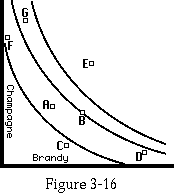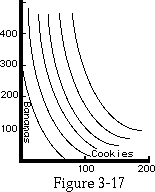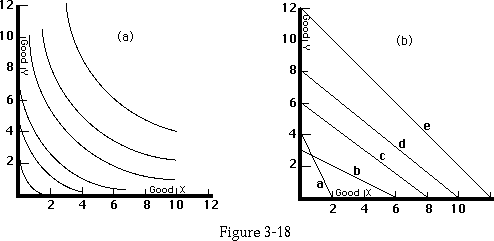7. Figures 3-19a, b, c, and d show four different sets of indifference curves; in each case, points on U3 are preferred to points on U2 , and points on U2 are preferred to points on U1. Describe verbally the pattern of preferences illustrated in each case. Yes, they are odd.

8. Figure 3-19e shows your preferences with regard to two goods--left shoes and right shoes. Explain why the indifference curves have the shape shown.

9. Draw a possible set of indifference curves for two things that are close, but not perfect, complements. An example might be bread and butter, if you much prefer your bread with butter but are willing to eat bread without butter (or with less than your preferred amount of butter).

10. Draw a possible set of indifference curves for two things that are perfect substitutes--butter and margarine for someone who cannot tell them apart. Draw another set for thwo things that are close, but not perfect, substitutes. An example might be chicken and turkey, if for some recipes you mildly prefer one or the other.

11. Figure 3-20 shows an indifference curve map and a budget line.

a. What is your marginal rate of substitution at points A, B, C ?

b. What is the slope of the budget line at points A, B, C ?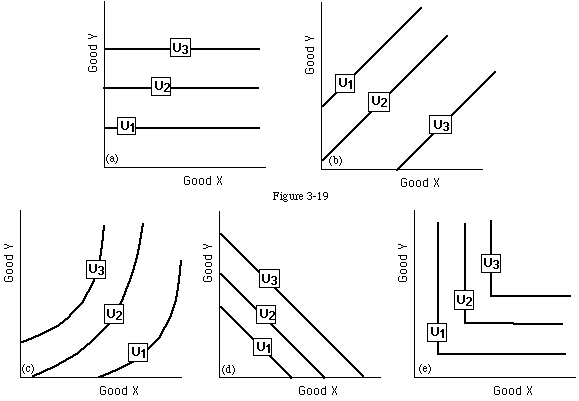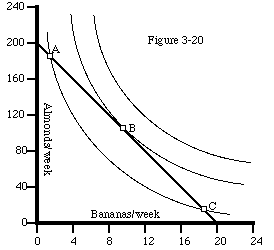12. Use Figure 3-4 to derive an income-adjusted demand curve for Apples; B1 on the figure should be one of your budget lines.

13. Use Figure 3-4 to derive an ordinary demand curve for Apples; assume that your income is \$100 and the price of oranges is \$1.

14. William's income is \$3/day; apples cost \$0.50/apple.

a. Draw William's budget line, showing the choice between apples and expenditure on all other goods.

b. In order to reduce medical expenditures, the government decides to subsidize apples; for every dollar William spends on apples, he will be given \$0.25 back. William pays no taxes. Draw William's budget line .

C. Instead of a subsidy, the government decides to use a voucher. The government provides William with \$0.50/day which can only be spent on apples; any part of it that he does not spend on apples must be returned. William still pays no taxes. Draw his budget line. Be careful; it will not look like the other budget lines we have drawn.

15. The situation is the same as in the previous problem. Figure 3-21 shows William's indifference curves. How many apples a day does William consume:

a. With neither subsidy nor voucher?

b. With subsidy?

c. With voucher?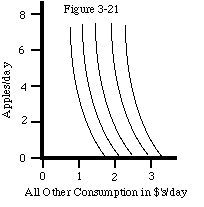16. Suppose that instead of subsidizing potatoes, as discussed in the text, we tax them; for every \$2 you spend on potatoes, you must give an additional \$1 to the government. The tax collected is then returned to the consumers as a demogrant: everyone gets a fixed number of dollars to add to his income. We assume that everyone has the same income and the same tastes.

Would people be better or worse off than if there were no tax (and no subsidy)? Prove your answer.

The following problems refer to the optional section:

17. What testable proposition is suggested by the statement "A has more utility than B to me?"

18. Do each of a-d, both geometrically (you need not be precise) and using calculus. There are only two goods; x is the quantity of one good and y of the other. Your income is I. u(x,y) = xy + x + y.

a. Px = \$1; Py = \$1; I = \$10. Suppose Py rises to \$2. By how much must I increase in order that you be as well off as before?

b. In the case described in part (a), assuming that I does not change, what quantities of each good are consumed before and after the price change? How much of each change is a substitution effect? How much is an income effect?

c. Px = \$1; I =\$10. Graph the amount of Y you consume as a function of Py , for values of Py ranging from \$0 to \$10 (your ordinary demand curve for Y).

d. With both prices equal to \$1, show how consumption of each good varies as I changes from \$0 to \$100.

19. Answer the following questions for the utility function:

u (x,y ) = x - 1/y

a. Px = \$1 ; I = \$10.Draw the demand curve for good Y, \$1 < Py < \$100.

b. Px = \$1 ; I = \$10.Py increases from \$1 to \$2. Show the old and the new equilibria. The income effect could be eliminated either by changing I or by changing Px and Py while keeping their ratio fixed. What would the necessary change in I be? What would the necessary change in the prices be? Diagram both.

c. Px = \$1. Draw the income-compensated demand curve for good Y. \$1 < Py < \$100. Start with Py=\$1 and I =\$10.

d. Px = \$1 = Py .Graph y against I for \$0 < I < \$10.

Next Chapter